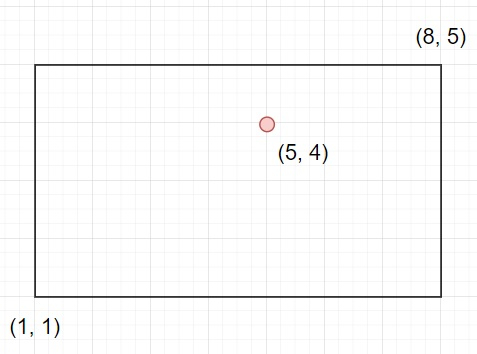# Check if a point lies on or inside a rectangle in Python

Suppose we have a rectangle represented by two points bottom-left and top-right corner points. We have to check whether a given point (x, y) is present inside this rectangle or not.

So, if the input is like bottom_left = (1, 1), top_right = (8, 5), point = (5, 4), then the output will be TrueTo solve this, we will follow these steps −

• Define a function solve() . This will take bl, tr, p
• if x of p > x of bl and x of p < x of tr and y of p > y of bl and y of p < y of tr, then
• return True
• otherwise,
• return False

Let us see the following implementation to get better understanding −

## Example

Live Demo

def solve(bl, tr, p) :
if (p > bl and p < tr and p > bl and p < tr) :
return True
else :
return False
bottom_left = (1, 1)
top_right = (8, 5)
point = (5, 4)
print(solve(bottom_left, top_right, point))

## Input

(1, 1), (8, 5), (5, 4)

## Output

True